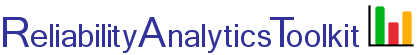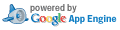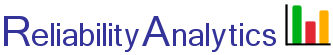# Reliability Growth Tracking

This tool provides the ability to plot and track reliability growth trends based on a series of failure times entered into box 2, one per line. It implements the AMSAA reliability growth model and statistical tests for trends, as published in MIL-HDBK-189 (Ref. 1). For several examples, skip inputs 1 - 4 and select one of the test sets (last input under item #5, Options).

## Calculation Inputs:

1. Total accumulated test time (unit-hours):

2. Failure times, one per line:

If multiple systems are tested, failure times are in units of cumulative unit-hours at each failure point. For example, two units are tested, unit 1 fails at 80 hours while unit 2 has accumulated 50 hours, the failure time is 130 unit-hours.
Example failure times

3. Significance levels:

a) For "no growth" test of hypothesis:
b) For Cramer-von Mises goodness of fit test:

4. Confidence level

5. Options:

Chart based on data fitted to AMSAA model
Chart based on calculated instantaneous value at each failure point
Calculate reliability at test time (unit-hours):

Decimal places: Apply to all.
Send results to:

Use a test data set (ignores inputs above):

## Featured Reference:Reliability: Management, Methods, and Mathematics

Toolkit Home